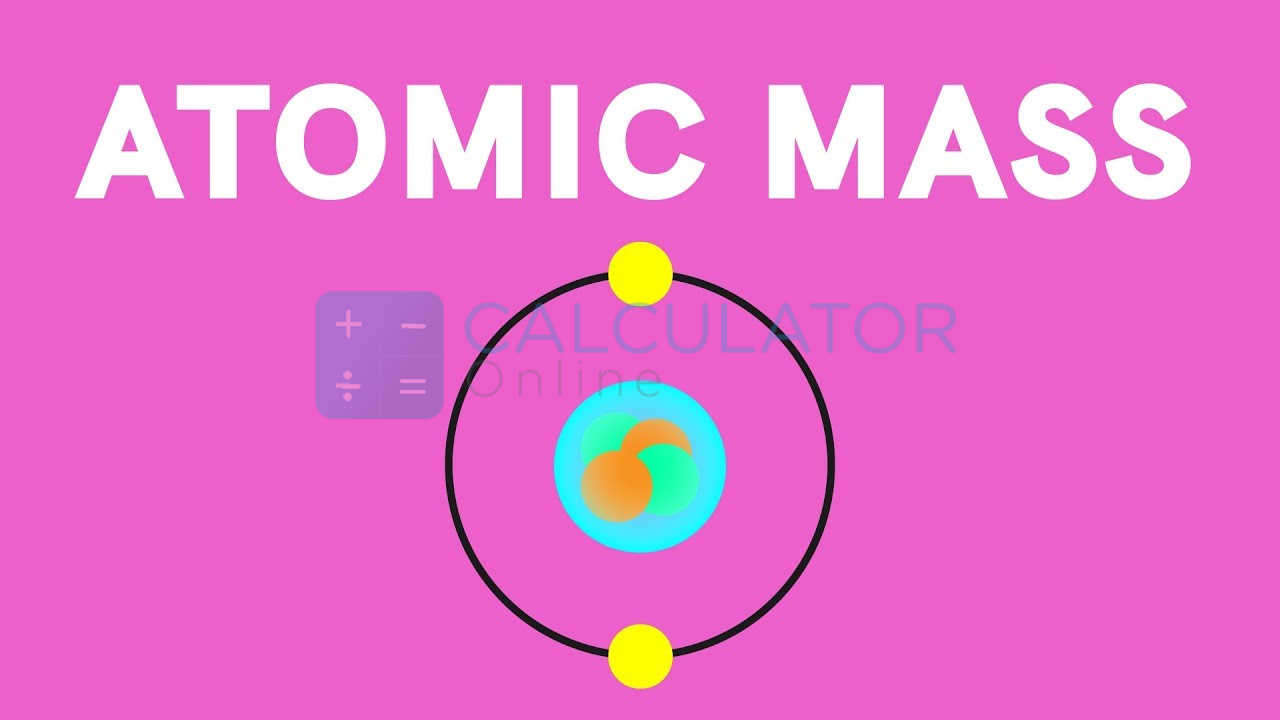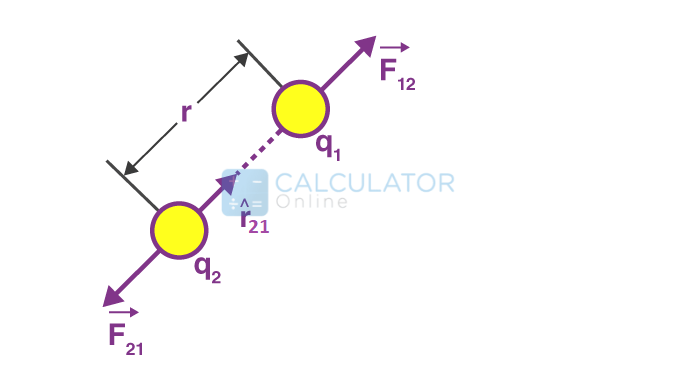• Hire UsUh Oh! It seems you’re using an Ad blocker!

We always struggled to serve you with the best online calculations, thus, there's a humble request to either disable the AD blocker or go with premium plans to use the AD-Free version for calculators.

Or# Atomic Mass of Elements

## What Is An Atom?

“The smallest unit in the element that contains all the chemical properties in it”### Parts of atoms:

A current model of atom can split into three constituents parts and each part is associated with a charge:
• Protons that carry positive charge
• Electrons that carry negative charge
• Neutrons that carry no net charge
According to the Standard Model of particle physics, protons and neutrons are present in the nucleus of the atom, while electrons revolve around the nucleus.
The electron configuration of an element explains how electrons are available in the atomic number of an atom. Whether you have multiple electrons in an atom, you can determine with the help of an electron configuration calculator.

## What is the atomic mass of elements?

The atomic mass of a given element can be defined as:
“The average mass of the atoms of a given element measured in the unified atomic mass unit (amu) is known as the atomic mass of an element”
• It is denoted by the symbol ‘u’.

### Unified atomic mass unit (amu)

Generally, one unified atomic mass unit can be evaluated as the weight of one-twelfth of the mass of a carbon-12 atom considered at rest which is also referred as relative atomic mass of elemente. Atomic masses of elements are equal to their mass number, since protons and neutrons have the same for all the mass for a given atom.

### How to calculate the atomic mass no of elements?

Standard atomic mass is determined by the average weight of all the isotopes of a given sample element. The average atomic mass of elements can be calculated by the abundance of each isotope multiplied by the mass number of elements.
For example:
Consider the gas neon, since it has three isotopes:
Neon-20:
• It has ten protons and ten neutrons in its nucleus. Therefore, the atomic mass unit of the neon gas is 19.992, and the abundance of the gas is 90.48%.
Neon-21:
• It has ten protons and eleven neutrons in its nucleus. Therefore the atomic mass unit of the neon gas is 20.994, and the abundance of the gas is 0.27%.
Neon-22:
• It has ten protons and twelve neutrons in its nucleus. The atomic mass unit of the neon gas is 21.991, and the abundance of the gas is 9.25%.
To calculate the atomic mass of neon, the average of the isotopes is required to be taken out:

0.9048 × 19.992 = 18.09 amu

0.0027 × 20.994 = 0.057 amu

0.0925 × 21.991 = 2.03 amu

18.09 +  0.057 + 2.03 = 20.18
The average atomic mass is thus: 20.18 amu

### The atomic mass of first 30 elements

Let’s discuss the atomic mass of elements 1 to 30 in the periodic table. Here you can also find out the atomic mass of first 20 elements with the help of the atomic mass calculator. Given below is an atomic mass table that lists that is based on atomic number and their corresponding atomic mass of all elements.
 ELEMENT ATOMIC MASS Hydrogen 1.008 Helium 4.0026 Lithium 6.94 Beryllium 9.0122 Boron 10.81 Carbon 12.011 Nitrogen 14.007 Oxygen 15.999 Fluorine 18.998 Neon 20.180 Sodium 22.990 Magnesium 24.305 Aluminium 26.982 Silicon 28.085 Phosphorus 30.974 Sulfur 32.06 Chlorine 35.45 Argon 39.948 Potassium 39.098 Calcium 40.078 Scandium 44.956 Titanium 47.867 Vanadium 50.942 Chromium 51.996 Manganese 54.938 Iron 55.845 Cobalt 58.933 Nickel 58.693 Copper 63.546 Zinc 65.38
Now, let us discuss atomic numbers and isotopes to clarify the above example.

## Atomic number

An atomic number of an element can be defined as:
“Number of protons in a neutral atom which is equal to number electrons”
Example:
An atom of chlorine has 17 protons in its nucleus; hence, the atomic number of chlorine is 17. And, atomic mass of elements is equal to 35.45 of chlorine.

### First 30 elements with atomic number

Let’s discuss the atomic number 1 to 30 in the periodic table. Look at the given table and describe the atomic number of elements from 1 to 30.
 ELEMENT ATOMIC NUMBER Hydrogen 1 Helium 2 Lithium 3 Beryllium 4 Boron 5 Carbon 6